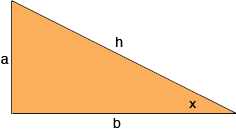SEARCH HOMEMath Central Quandaries & QueriesQuestion from Mubashir, a student: there are two curves y=sinx and y=cosx intersecting at point where x is less than 90 and greater than 0.to find the point I made a equation sinx=cosx but do not know what to do further .please help me by solving this equation sinx=cosx also tell me that have you solved this equation by reference to the graph or by other means please do elaborate your solution in your answer.Hi Mubashir,

Suppose you have a right triangle with sides of length $a, b, \mbox{ and }h$ units where $h$ is the length of the hypotenuse. Suppose one of the angles of the triangle has measure $x$ degrees as in the diagram.What is the sine of $x$ in terms of $a, b, \mbox{ and }h?$ What is the cosine of $x$ in terms of $a, b, \mbox{ and }h?$ If the sine and cosine are equal what can you say about $a$ and $b?$ What is $x?$

PennyMath Central is supported by the University of Regina and the Imperial Oil Foundation.